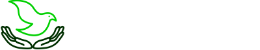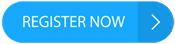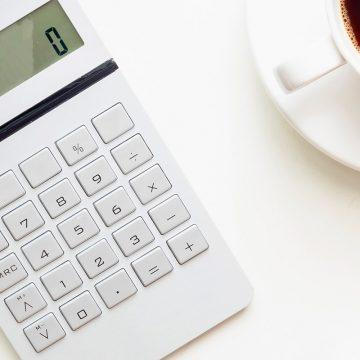×InvestingStocksToolsClubsVideosPrivacy PolicyTerms And Conditions
Subscribe To Our Newsletter# How to use the Interest Compounding Calculator

||You have probably already heard of the compound interest calculator. Let's take a closer look at this tool. Let's find out what it is, where and how it is used, and look at examples of its use.

## What is compound interest?

Compound interest in finance is interest income accrued on the amount of deposits or investments, taking into account interest previously accumulated for previous periods. Financiers use another term, capitalisation of interest. Although the field of application of compound interest is much broader than capital accumulation, it is still the most popular in this segment.

Compound interest on a deposit with capitalisation may be calculated daily, monthly, quarterly and annually. If they are not paid out, they are added to the deposit amount and interest will accrue on a larger amount in the next period. Thus, the essence of compound interest is that each time the calculation base increases.

This scheme is also sometimes called "interest on interest". Compound interest multiplies capital at an accelerated rate. The longer the periods in which it is compounded, the greater the return. Combining compound interest with regular investments over a long period of time is a highly effective way of preserving and increasing capital.

## Why use a compound interest calculator?

The calculation of compound interest (income accruing with constant reinvestment) is a complex mathematical operation. The formula for the calculation is as follows:

A = P(1+r/n)^nt

In the formula:

• A is the future amount of capital, including accrued interest.
• P is the principal amount of the investment.
• r is the interest rate (decimal).
• n is the number of interest accruals per period.
• t is the number of periods for which funds are invested.

The formula is not the easiest to calculate, so if you're afraid of making a mistake, you'd better use a special tool: a compound interest calculator.

If you are not afraid of error and only need to calculate an approximate return on compound interest, the "Rule of 72" can help. We talked about it earlier in one of our articles.

However, for an accurate and detailed calculation, it is recommended that you use the above tool. It is essentially an investment calculator that helps to calculate the potential return on an investment. It can be used to calculate daily, monthly or yearly interest. It is a versatile tool that can be used in many ways, including as a forex interest calculator.

## Using the compound interest calculator

There are many websites with different online calculators available on the internet. Let's take the tool from https://www.thecalculatorsite.com as an example

The following parameters must be specified for the calculation:

• The currency of the investment.
• The amount of investment.
• Interest rate (daily, weekly, monthly, annual).
• Investment term.
• The periodicity of compound interest.

If necessary, additional parameters can be entered:

• Regularly depositing funds.
• Regular withdrawals.
• Contribution of funds to take account of annual inflation.

An example of using the compound interest calculator

Let's calculate what the financial result would be if the funds were invested under these conditions:

• Deposit currency: USD.
• Investment amount: 5000 USD.
• Interest rate: 1% per month.
• Investment period: 5 years.
• Compound interest accrual: monthly.

Enter the above data into the calculator and press the "Calculate" button. The result is a detailed calculation in the form of a table and a graph, which shows that after 5 years the capital will be 9 083 USD. That is, the initial investment, thanks to the reinvestment of profits, will almost double over that time.

If simple interest is calculated under the same conditions, the total will be 8 000 USD. As you can see, the additional interest income increases the profit considerably. The duration of the investment has a big influence on the total amount: for short-term programmes (less than one year), the benefit of compound interest is hardly significant.

## Conclusion

Compound interest is a great way to multiply capital. It is particularly relevant in a long-term context. An investor can use a calculator to calculate the expected financial result.

With this tool, you can examine your investment prospects in detail, taking into account a number of individual parameters, such as the amount invested, the interest rate or the term of the investment.

The post How to Use Interest Compounding Calculator appeared first at R Blog - RoboForex.

||

-----------------------------------------------------

By: Victor Gryazin
Title: How to Use Interest Compounding Calculator
Sourced From: blog.roboforex.com/blog/2022/10/21/how-to-use-interest-compounding-calculator/
Published Date: Fri, 21 Oct 2022 15:32:20 +0000